# Setup for 5 Player a Game of Food Chain Magnate

Originally generated on 1/11/2019 1:03:55 AM
A direct link to this setup is http://www.VentersConsulting.com/BoardgameHelpers/FoodChainMagnateGenerator.aspx?LoadGame=\$2a\$04\$ApDV3DMxkpETZJixjiwMme

## Player Setup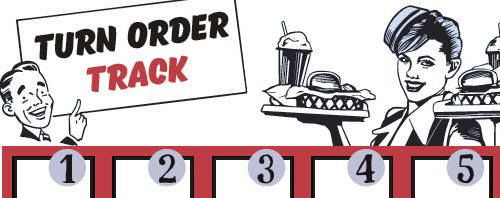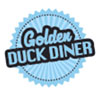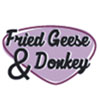## Other Setup

Bank starts with \$250.00
Number of 1x Employee cards used = 3

## Map (The Way Out Suburbs)

Click here to view Map Tile Lettering Key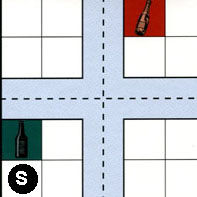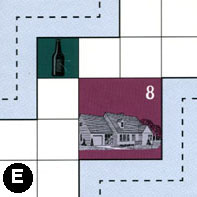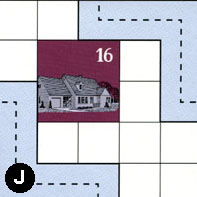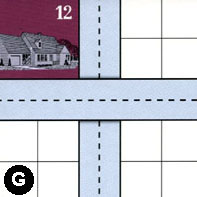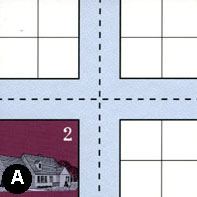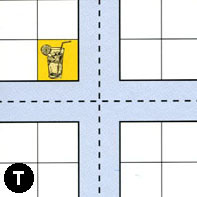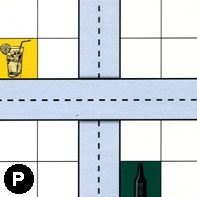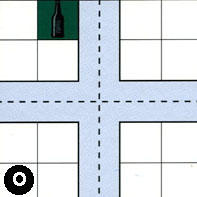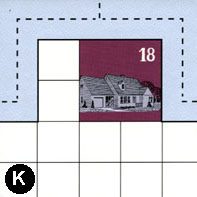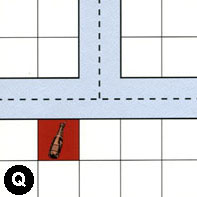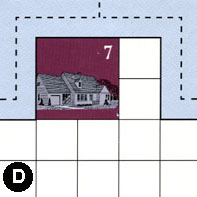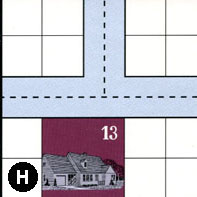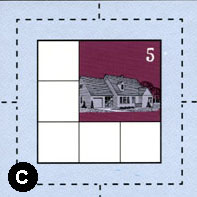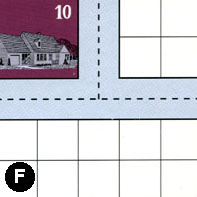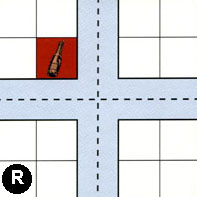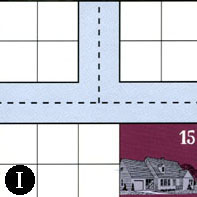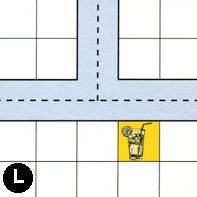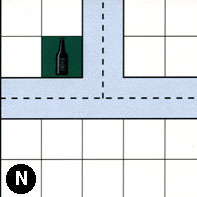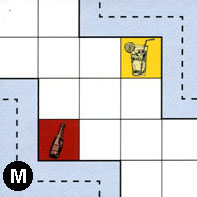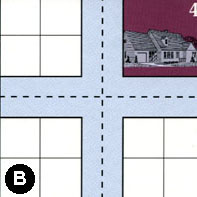### Map Stats

Map Option = The Way Out Suburbs
Total Number of Tiles = 20
Total Number of Starting Houses = 11
Total Number of Beer Spots = 5
Total Number of Soda Spots = 4
Total Number of Lemonade Spots = 4
Total Number of Drink Spots = 13
Number of independent path systems = 1
Number of independent neighborhoods = 21

Path System 1
Size = Huge
Number of Tiles = 20
Number of Paths = 76
Contains Loop(s) = No
Number of Starting Houses = 12
Starting Houses Pct of Map (%) = 109
Number of Beer Spots = 5
Number of Soda Spots = 4
Number of Lemonade Spots = 4
Number of Drink Spots = 13
Drink Spots Pct of Map (%) = 100
Tile Ids = A, B, C, D, E, F, G, H, I, J, K, L, M, N, O, P, Q, R, S, T
Paths = SWS, EES, SWN, TWN, TWE, PNS, OWE, KWN, FWN, RWN, QWN, QNE, AWE, AWN, ANE, QWE, RNE, RWE, FNE, MEN, FWE, KWE, GNS, KNE, OWN, CWS, CWN, NWE, NWN, LWE, IWN, INE, IWE, LWN, HWN, HNE, PWE, EWN, JWS, OWS, ONS, CNS, NNE, LNE, HWE, CNE, CWE, CES, ONE, OES, RWS, BWN, BNE, BWE, RNS, RES, BWS, MWS, BNS, BES, AES, GWE, JEN, AWS, ANS, TNE, SNE, SWE, SES, SNS, TNS, DWE, DWN, DNE, TWS, TES What do these mean?
Starting House Ids = 0, 2, 4, 5, 7, 8, 10, 12, 13, 15, 16, 18

Neighborhood 1
Total Size = Small
Number of Total Spaces = 4
Number of Empty Spaces (for new houses & gardens) = 4
Number of Beer Spots = 0
Number of Soda Spots = 0
Number of Lemonade Spots = 0
Number of Drink Spots = 0
Number of Starting Houses = 0
Starting Houses Pct of Map (%) = 0
Tile Ids = S
Neighborhood 2
Total Size = Small
Number of Total Spaces = 4
Number of Empty Spaces (for new houses & gardens) = 3
Number of Beer Spots = 1
Number of Soda Spots = 0
Number of Lemonade Spots = 0
Number of Drink Spots = 1
Number of Starting Houses = 0
Starting Houses Pct of Map (%) = 0
Tile Ids = S
Neighborhood 3
Total Size = Large
Number of Total Spaces = 50
Number of Empty Spaces (for new houses & gardens) = 41
Number of Beer Spots = 1
Number of Soda Spots = 0
Number of Lemonade Spots = 0
Number of Drink Spots = 1
Number of Starting Houses = 2
Starting Houses Pct of Map (%) = 18
Tile Ids = E, G, J, O, P, S, T
Starting House Ids = 8, 16
Neighborhood 4
Total Size = Small
Number of Total Spaces = 4
Number of Empty Spaces (for new houses & gardens) = 4
Number of Beer Spots = 0
Number of Soda Spots = 0
Number of Lemonade Spots = 0
Number of Drink Spots = 0
Number of Starting Houses = 0
Starting Houses Pct of Map (%) = 0
Tile Ids = G
Neighborhood 5
Total Size = Medium
Number of Total Spaces = 8
Number of Empty Spaces (for new houses & gardens) = 4
Number of Beer Spots = 0
Number of Soda Spots = 0
Number of Lemonade Spots = 0
Number of Drink Spots = 0
Number of Starting Houses = 1
Starting Houses Pct of Map (%) = 9
Tile Ids = A, G
Starting House Ids = 2
Neighborhood 6
Total Size = Small
Number of Total Spaces = 4
Number of Empty Spaces (for new houses & gardens) = 4
Number of Beer Spots = 0
Number of Soda Spots = 0
Number of Lemonade Spots = 0
Number of Drink Spots = 0
Number of Starting Houses = 0
Starting Houses Pct of Map (%) = 0
Tile Ids = A
Neighborhood 7
Total Size = Medium
Number of Total Spaces = 8
Number of Empty Spaces (for new houses & gardens) = 6
Number of Beer Spots = 0
Number of Soda Spots = 1
Number of Lemonade Spots = 1
Number of Drink Spots = 2
Number of Starting Houses = 0
Starting Houses Pct of Map (%) = 0
Tile Ids = S, T
Neighborhood 8
Total Size = Large
Number of Total Spaces = 40
Number of Empty Spaces (for new houses & gardens) = 27
Number of Beer Spots = 0
Number of Soda Spots = 1
Number of Lemonade Spots = 0
Number of Drink Spots = 1
Number of Starting Houses = 3
Starting Houses Pct of Map (%) = 27
Tile Ids = A, F, G, K, Q, R
Starting House Ids = 10, 12, 18
Neighborhood 9
Total Size = Medium
Number of Total Spaces = 18
Number of Empty Spaces (for new houses & gardens) = 17
Number of Beer Spots = 0
Number of Soda Spots = 1
Number of Lemonade Spots = 0
Number of Drink Spots = 1
Number of Starting Houses = 0
Starting Houses Pct of Map (%) = 0
Tile Ids = A, Q, R
Neighborhood 10
Total Size = Medium
Number of Total Spaces = 8
Number of Empty Spaces (for new houses & gardens) = 7
Number of Beer Spots = 1
Number of Soda Spots = 0
Number of Lemonade Spots = 0
Number of Drink Spots = 1
Number of Starting Houses = 0
Starting Houses Pct of Map (%) = 0
Tile Ids = O, P
Neighborhood 11
Total Size = Small
Number of Total Spaces = 4
Number of Empty Spaces (for new houses & gardens) = 4
Number of Beer Spots = 0
Number of Soda Spots = 0
Number of Lemonade Spots = 0
Number of Drink Spots = 0
Number of Starting Houses = 0
Starting Houses Pct of Map (%) = 0
Tile Ids = T
Neighborhood 12
Total Size = Large
Number of Total Spaces = 46
Number of Empty Spaces (for new houses & gardens) = 37
Number of Beer Spots = 0
Number of Soda Spots = 0
Number of Lemonade Spots = 1
Number of Drink Spots = 1
Number of Starting Houses = 2
Starting Houses Pct of Map (%) = 18
Tile Ids = D, H, I, L, P, T
Starting House Ids = 7, 15
Neighborhood 13
Total Size = Large
Number of Total Spaces = 26
Number of Empty Spaces (for new houses & gardens) = 22
Number of Beer Spots = 0
Number of Soda Spots = 0
Number of Lemonade Spots = 0
Number of Drink Spots = 0
Number of Starting Houses = 1
Starting Houses Pct of Map (%) = 9
Tile Ids = H, L, N, O, P
Starting House Ids = 13
Neighborhood 14
Total Size = Medium
Number of Total Spaces = 14
Number of Empty Spaces (for new houses & gardens) = 13
Number of Beer Spots = 1
Number of Soda Spots = 0
Number of Lemonade Spots = 0
Number of Drink Spots = 1
Number of Starting Houses = 0
Starting Houses Pct of Map (%) = 0
Tile Ids = F, O
Neighborhood 15
Total Size = Medium
Number of Total Spaces = 9
Number of Empty Spaces (for new houses & gardens) = 5
Number of Beer Spots = 0
Number of Soda Spots = 0
Number of Lemonade Spots = 0
Number of Drink Spots = 0
Number of Starting Houses = 1
Starting Houses Pct of Map (%) = 9
Tile Ids = C
Starting House Ids = 5
Neighborhood 16
Total Size = Large
Number of Total Spaces = 51
Number of Empty Spaces (for new houses & gardens) = 48
Number of Beer Spots = 1
Number of Soda Spots = 1
Number of Lemonade Spots = 1
Number of Drink Spots = 3
Number of Starting Houses = 0
Starting Houses Pct of Map (%) = 0
Tile Ids = B, F, M, N, O, R
Neighborhood 17
Total Size = Medium
Number of Total Spaces = 8
Number of Empty Spaces (for new houses & gardens) = 8
Number of Beer Spots = 0
Number of Soda Spots = 0
Number of Lemonade Spots = 0
Number of Drink Spots = 0
Number of Starting Houses = 0
Starting Houses Pct of Map (%) = 0
Tile Ids = B, R
Neighborhood 18
Total Size = Small
Number of Total Spaces = 4
Number of Empty Spaces (for new houses & gardens) = 4
Number of Beer Spots = 0
Number of Soda Spots = 0
Number of Lemonade Spots = 0
Number of Drink Spots = 0
Number of Starting Houses = 0
Starting Houses Pct of Map (%) = 0
Tile Ids = I
Neighborhood 19
Total Size = Medium
Number of Total Spaces = 18
Number of Empty Spaces (for new houses & gardens) = 16
Number of Beer Spots = 1
Number of Soda Spots = 0
Number of Lemonade Spots = 1
Number of Drink Spots = 2
Number of Starting Houses = 0
Starting Houses Pct of Map (%) = 0
Tile Ids = I, L, N
Neighborhood 20
Total Size = Small
Number of Total Spaces = 4
Number of Empty Spaces (for new houses & gardens) = 4
Number of Beer Spots = 0
Number of Soda Spots = 0
Number of Lemonade Spots = 0
Number of Drink Spots = 0
Number of Starting Houses = 0
Starting Houses Pct of Map (%) = 0
Tile Ids = B
Neighborhood 21
Total Size = Small
Number of Total Spaces = 4
Number of Empty Spaces (for new houses & gardens) = 0
Number of Beer Spots = 0
Number of Soda Spots = 0
Number of Lemonade Spots = 0
Number of Drink Spots = 0
Number of Starting Houses = 1
Starting Houses Pct of Map (%) = 9
Tile Ids = B
Starting House Ids = 4

v8.3.2